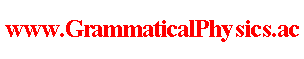since 2006
Help　Sitemap
< Forum >
< Problems >
< Quantum History Theory >
< Uda Equation of Dirac Field >

At first, I note down the every component of the matrices to be used.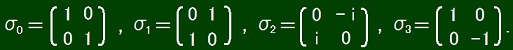σ1σ2 = -σ2σ1 = iσ3, σ2σ3 = -σ3σ2 = iσ1, σ3σ1 = -σ1σ3 = iσ2,
σ1σ1 = σ2σ2 = σ3σ3 = σ0.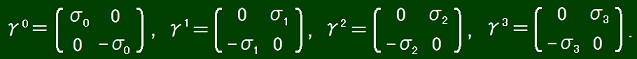μ, γν]+ = γμγν + γνγμ = 2gμν,
g00 = 1, g11 = g22 = g33 = -1, μ≠ν ⇒ gμν = 0.
When I was studying the Dirac equation, I did not think of noting down these details fully as necessary because I thought of such things as obvious.
So, now, a few decades after that, it has taken much effort for me to extract the above information.

The Hamiltonian H of the Dirac Field is as follows.
H = ∫d3x ψ†(x0(-iγkk + m)ψ(x)
Here (γ0γk)† = γk†γ0† = (-γk)(γ0) = -γkγ0 = γ0γk that is to say hermitian.
The quantization condition is as follows.
α(x), ψβ†(y)]+ = δαβδ3(x - y),
α(x), ψβ(y)]+ = 0.
The Schrodinger equation is as follows.
ih(d/dt)|ΨS(t)> = H|ΨS(t)>.

As a preparation, let's consider a quantum mechanics of two real degrees x, y of freedom.
H = c(x - iy)(x + iy),
[x + iy, x - iy]+ ≡ (x + iy)(x - iy) + (x - iy)(x + iy) = 1,
[x + iy, x + iy]+ ≡ (x + iy)(x + iy) + (x + iy)(x + iy) = 0.
ih(d/dt)|ΨS(t)> = H|ΨS(t)>
where c is real.

(x + iy)(x - iy) + (x - iy)(x + iy) = 2x2 + 2y2
∴ x2 + y2 = 1/2

(x + iy)(x + iy) = x2 - y2 + i(xy + yx)
∴ [x, y]+ = i(x2 - y2) = i(2x2 - 1/2) = i(1/2 - 2y2)

a ≡ x + iy, [a, a†]+ = 1, [a, a]+ = 0.

[a†a, a]- = a†aa - aa†a = -aa†a = -(1 - a†a)a = -a,
[a†a, a†]- = a†aa† - a†a†a = a†aa† = (1 - aa†)a† = a†.

a†a|n> = n|n>, <n|n> = 1.

a†aa|n> = 0 = 0a|n>　∵ aa = 0
(a|n>, a|n>) = <n|a†a|n> = n<n|n> = n
∴ a|n> = (√n)|0>

a†aa†|n> = (a†a†a + [a†a, a†]-)|n> = [a†a, a†]-|n> = a†|n> = 1a†|n>,
(a†|n>, a†|n>) = <n|aa†|n> = <n|(1 - a†a)|n> = (1 - n)<n|n> = 1 - n
∴ a†|n> = [√(1 - n)]|1>

n2<n|n> = (a†a|n>, a†a|n>) = <n|a†aa†a|n> = <n|a†(1 - a†a)a|n> = <n|a†a|n> = n<n|n>
∴ n2 = n
∴ n(n - 1) = 0
∴ n = 0 or 1.

a|0> = 0, a|1> = |0>, a†|0> = |1>, a†|1> = 0.

This is possible because there exsists the following concrete representation.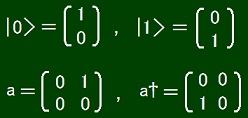|0> and |1> are the following functions.
φ0(0) = 1 and φ0(1) = 0 ・・・ |0>
φ1(0) = 0 and φ1(1) = 1 ・・・ |1>

a and a† are the following linear operators.
0 = 0 and aφ1 = φ0,
a†φ0 = φ1 and a†φ1 = 0.

An arbitrary function φ is expressed as
φ = φ(0)φ0 + φ(1)φ1
and so
aφ = φ(0)aφ0 + φ(1)aφ1 = φ(1)φ0,
a†φ = φ(0)a†φ0 + φ(1)a†φ1 = φ(0)φ1,
a†aφ = [aφ(0)]φ1 = φ(1)φ0(0)φ1 = φ(1)φ1
∴ (aφ)(n) = φ(1)φ0(n) = δn0Σk=01δk1φ(k),
(a†φ)(n) = φ(0)φ1(n) = δn1Σk=01δk0φ(k),
(a†aφ)(n) = φ(1)φ1(n) = δn1φ(1) = δn1φ(n).

A quantum history is represented by a functional Φ whose variable is a function n and whose value is a complex number.
Φ[n] ∈ C,
n(t) = 0 or 1,
t ∈ R.

ih limε→+0(1/ε){Φ[n(□ -ε)] - Φ[n]} = cα∫-∞dt δn(t),1Φ[n].

tΦ[n] ≡ limε→+0(1/ε){Φ[n'] - Φ[n'']},
t - ε≦ t' ＜ t ⇒ n'(t') = 1 and n''(t') = 0,
t' ＜ t - ε or t' ≧ t ⇒ n'(t') = n''(t') = n(t').

Φ[n(□ -ε)] - Φ[n] ～ -ε∫-∞dt [dn(t)/dt]⊿tΦ[n]
because dn(t)/dt is a sum of delta functions.

-ih-∞dt [dn(t)/dt]⊿tΦ[n] = cα∫-∞dt δn(t),1Φ[n].
∴∫-∞dt {ih[dn(t)/dt]⊿t + cαδn(t),1]Φ[n]
witten at 2019/04/18/14:57.

On the other hand, the Schrodinger equation of the same system in the old grammar is derived as follows.

ih(∂/∂t)[φ(0, t)|0> + φ(1, t)|1>] = ca†a[φ(0, t)|0> + φ(1, t)|1>]
∴ ih(∂/∂t)φ(0, t)|0> + ih(∂/∂t)φ(1, t)|1> = cφ(1, t)|1>
∴ ih(∂/∂t)φ(0, t) = 0 and ih(∂/∂t)φ(1, t) = cφ(1, t).

A solution of this set of equations must be as follows.
φ(0, t) = c1, φ(1, t) = c2 exp[-(i/h)ct].
The normalization condition fort this solution is as follows.
|φ(0, t)|2 + |φ(1, t)|2 = 1
∴ |c1|2 + |c2|2 = 1.
I am glad of how plausible this solution is.

-ih-∞dt [dn(t)/dt][⊿/⊿n(t)]Φ[n] = cα∫-∞dt n(t)Φ[n]
where [⊿/⊿n(t)]Φ[n]≡ limε→+0(1/ε){Φ[n'] - Φ[n'']},
t - ε≦ t' ＜ t ⇒ n'(t') = 1 and n''(t') = 0,
t' ＜ t - ε or t' ≧ t ⇒ n'(t') = n''(t') = n(t').
This is a good conclusion for the preparation system.
However, another construction may be possible.
It is as follows.
[a(t), a†(t')]+ = δ(t - t'),
ih limε→+0(1/ε){Φ[ν(□ -ε)] - Φ[ν]} = cα∫-∞dt a†(t)a(t)Φ[ν].
This method also realize [a†(t)a(t), a†(t')a(t')]- = 0 if t ≠ t'.
If I use this method, the eigenvalue ν(t) of a†(t)a(t) is not finite but δ(0).
I want to avoid using such ν.
As for the above preparation system, I can adopt the method using n(t) = 0, 1 described above.
However, for the Dirac field as our goal, the eigenvalue of ψα†(xα(x) is not finite but δ3(0).
So, for the Dirac field, I can not adopt the method using n(t) = 0, 1 described above.
Moreover, [a(t), a†(t')]+ = δ(t - t') fits [ψα(xβ†(y)]+ = δαβδ3(x - y) very well.
These conditions can be unified to a four-dimensional form:
α(x, t)ψβ†(y, t')]+ = δαβδ3(x - y)δ(t - t')
or [ψα(x)ψβ†(y)]+ = δαβδ4(x - y).
However, the number of fermions is countable at each time.
This does not fit the fact that the graph of n(t) is drawn only by lines.
To make such graph of n(t), we must let a†(t) operate to the vacuum for continuous interval of t.
We do not yet have a way to write it mathematically.2019/05/02/21:10

A good idea has arised.
Let us define |n> by the following condition.
n(t) = 0 ⇒ a(t)|n> = 0,
n(t) = 1 ⇒ a†(t)|n> = 0.
This definition enables n(t) to be 1 for all t in any continuous part of time axis.
However, a†(t)a(t)|n> = δ(0)|n> if n(t) = 1.
I do not want to use the infinite divergent factor δ(0) which is the value of the delta function at the origin.
Though it is one of eigenvalues of ψα†(xα(x) in QED, perhaps many theorists do not think of such a factor as acceptable.
So, I try replacing a†(t)a(t)|n> with n(t)|n>.
This prescription brings the following treatment to the Dirac field.
α(x, t)ψβ†(y, t')]+ = δαβδ3(x - y)δ(t - t'),
ρ(x, α, t) = 0 ⇒ ψα(x, t)|ρ> = 0,
ρ(y, α, t) = δ3(y - x) ⇒ ψα†(x, t)|ρ> = 0,
ψα†(x, t)ψα(x, t)|ρ> is replaced by ρ(x, α, t)|ρ>.
Here ρ should be understood as a three-dimensional number density.2019/05/03/19:14

ρ(x, α, t) = Σk δα, g(t, k)δ(x1 - f1(t, k))δ(x2 - f2(t, k))δ(x3 - f3(t, k)),
g(t, k) = 0 or 1 or 2 or 3 or 4.
δα, g(t, k) is not zero for some α only when t obeys g(t, k) ≠ 0.
ψα†(x, t)ψα(x, t)|ρ> = ρ(x, α, t)|ρ>.
ψα†(x, t)∂1ψβ(x, t)|ρ> = limε→+0(1/ε)(|ρ'> - |ρ''>),
ρ'(x', α', t') = Σk δα', g'(t', k)δ(x'1 - f'1(t', k))δ(x'2 - f2(t', k))δ(x'3 - f3(t', k)),
ρ''(x', α', t') = Σk δα', g'(t', k)δ(x'1 - f''1(t', k))δ(x'2 - f2(t', k))δ(x'3 - f3(t', k)),
g(t, k) ≠ β ⇒ g'(t', k) = g(t', k) and f'1(t', k) = f1(t', k).
g(t, k) = β ⇒ [t - ε' ≦ t' ＜ t ⇒ g'(t', k) = α] and [t' ＜ t - ε' or t' ≧ t ⇒ g'(t', k) = g(t', k)].
g(t, k) = β ⇒ [t' ＜ t - ε' or t' ≧ t ⇒ f'1(t', k) = f1(t', k)].
g(t, k) = β ⇒ [t' ＜ t - ε' or t' ≧ t ⇒ f''1(t', k) = f1(t', k)].
limε→+0ε' → 0.
The area between f'1(t', k) and f''1(t', k) is ε in t-f1 plane.
This idea is difficult to explain linguistically but graphically simple.2019/05/04/21:14

I give graphical representations below.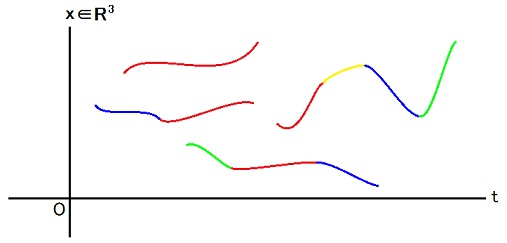This picture represents the number density function ρ such that
ρ(x, α, t) = Σk δα, g(t, k)δ(x1 - f1(t, k))δ(x2 - f2(t, k))δ(x3 - f3(t, k)),
g(t, k) = 0 or 1 or 2 or 3 or 4.
I want to write fk1(t), fk2(t), fk3(t), gk(t) in place of f1(t, k), f2(t, k), f3(t, k), g(t, k).
To avoid halving the size of gk(t), I have to write g(t, k).
Because I write g(t, k), I should write f1(t, k), f2(t, k), f3(t, k).
k is a name of a curve in the picture.
The k-th curve is expressed by the following set of three equations.
x1 = fk1(t), x2 = fk2(t), x3 = fk3(t).
gk(t) corresponds to the color of the point (t, x1, x2, x3) = (t, fk1(t), fk2(t), fk3(t)) and represents what fermion exists at the point.
If ψα†(fk1(t), fk2(t), fk3(t), t)|ρ> = 0, gk(t) = α.
 α color in picture 1 red 2 yellow 3 green 4 blue
I have set gk(t) = 0 for the purpose of terminating the k-th curve.
It works because α≠0.
Please notice that there can be more than one k for a same f because g can differ.
For example,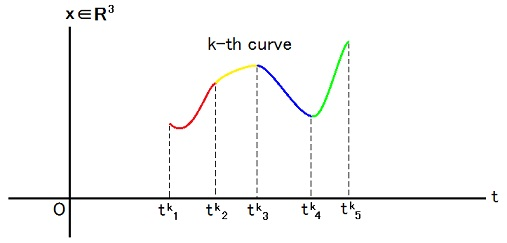t ＜ tk1 ⇒ gk(t) = 0,
tk1 ＜ t ＜ tk2 ⇒ gk(t) = 1,
tk2 ＜ t ＜ tk3 ⇒ gk(t) = 2,
tk3 ＜ t ＜ tk4 ⇒ gk(t) = 4,
tk4 ＜ t ＜ tk5 ⇒ gk(t) = 3,
t ＞ tk5 ⇒ gk(t) = 0.

∫d3x ψ4†(x, a)∂2ψ1(x, a)|ρ> ≡ limε→+0 (1/ε)(|ρ'> - |ρ''>),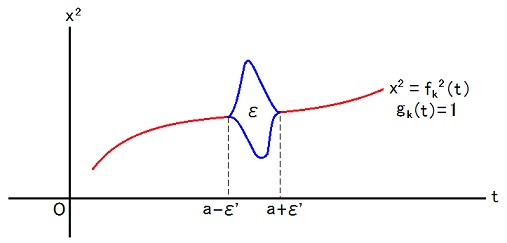ρ' is made by replacing every a -ε' < t < a +ε' part of each red curve with lower blue curve.
ρ'' is made by replacing every a -ε' < t < a +ε' part of each red curve with upper blue curve.
Only fk2 is deformed and neither fk3 nor fk1 is deformed.
gk(t) is changed from 1 to 4 only in the part a -ε' < t < a +ε'.
The area between two blue curves has to be ε.
ε' has to be positive and has to tend to zero as ε tends to zero.
As a definition, limit is taken just after replacement for each curve then all results of them are summed.
However, if we replace all necessary parts before subtraction and limitation, the result becomes same because of the character of derivative.2019/05/05/17:55.

f1(t) is a mapping which maps k on fk1(t),
f2(t) is a mapping which maps k on fk2(t),
f3(t) is a mapping which maps k on fk3(t),
g(t) is a mapping which maps k on gk(t),
f(t) is a mapping which maps j on fj(t),
f is a mapping which maps t on f(t),
g is a mapping which maps t on g(t),
ρ[ f, g ] ∈ R3×{1, 2, 3, 4}×RR.
{ ρ[ f, g ] }(x, α, t) ≡ Σk δ(α, gk(t))δ(x1 - fk1(t))δ(x2 - fk2(t))δ(x3 - fk3(t))
where δ(α, β) ≡ δαβ.
{
δαβ/[δfj(t)] } |ρ[ f, g ] > ≡ ∫d3x ψα†(x, t)∂jψβ(x, t) |ρ[ f, g ] > defined graphically above.

[ { ρ[ f, g ] }(□, □, □ - ε) ] (x, α, t) = { ρ[ f, g ] } (x, α, t - ε)
= Σk δ(α, gk(t - ε))δ(x1 - fk1(t - ε))δ(x2 - fk2(t - ε))δ(x3 - fk3(t - ε))
= { ρ[ f(□ - ε), g(□ - ε) ] } (x, α, t)
∴ { ρ[ f, g ] }(□, □, □ - ε) = ρ[ f(□ - ε), g(□ - ε) ].

limε→+0 (1/ε) [ | {ρ[ f, g ] } (□, □, □ - ε) > - |ρ[ f, g ] > ].
= -∫-∞dt Σkj=13[dfkj(t)/dt][δ/δfkj(t)] + [dgk(t)/dt][⊿/⊿gk(t)] }|ρ[ f, g ] >.

Uda Equation:
-ih-∞dt Σkj=13[dfkj(t)/dt][δ/δfkj(t)] + [dgk(t)/dt][⊿/⊿gk(t)] } Φ[ f, g ]
= α∫-∞dt {α=14Σβ=140γj)αβ { δαβ/[δfj(t)] }
+ m∫d3x [ {ρ[ f, g ] }(x, 1, t) + {ρ[ f, g ] }(x, 2, t) - {ρ[ f, g ] }(x, 3, t) - {ρ[ f, g ] }(x, 4, t) ] }.2019/05/06/17:40

In the above expression, I missed the last factor.
The first term of the integrand in the right hand side of the above equation has the opposite sign to the sign of the corresponding term in the original Hamiltonian.
This is not a mistake because partial integration was done.
The corrected expression of Uda equation of the free Dirac field is as follows.

-ih-∞dt Σkj=13[dfkj(t)/dt][δ/δfkj(t)] + [dgk(t)/dt][⊿/⊿gk(t)] } Φ[ f, g ]
= α∫-∞dt {j=13Σα=14Σβ=140γj)αβ { δαβ/[δfj(t)] }
+ m∫d3x [ {ρ[ f, g ] }(x, 1, t) + {ρ[ f, g ] }(x, 2, t)
- {ρ[ f, g ] }(x, 3, t) - {ρ[ f, g ] }(x, 4, t) ] }Φ[ f, g ].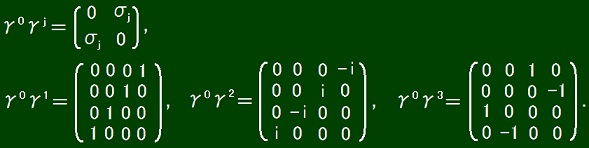By using these components of the matrices, Uda equation of the free Dirac field is written as follows.

-ih-∞dt Σkj=13[dfkj(t)/dt][δ/δfkj(t)] + [dgk(t)/dt][⊿/⊿gk(t)] } Φ[ f, g ]
= α∫-∞dt {14/[δf1(t)] + iδ23/[δf1(t)] + iδ32/[δf1(t)] + iδ41/[δf1(t)]
+ δ14/[δf2(t)] - δ23/[δf2(t)] + δ32/[δf2(t)] - δ41/[δf2(t)]
+ iδ13/[δf3(t)] - iδ24/[δf3(t)] + iδ31/[δf3(t)] - iδ42/[δf3(t)]
+ m∫d3x [ {ρ[ f, g ] }(x, 1, t) + {ρ[ f, g ] }(x, 2, t)
- {ρ[ f, g ] }(x, 3, t) - {ρ[ f, g ] }(x, 4, t) ] }Φ[ f, g ].
2019/05/07/15:20.

The above expression still includes mistakes.
ΣρΦ[ρ] ( |ρ'> - |ρ''> ) = Σρ{ Φ[ 'ρ] - Φ[ ''ρ] } |ρ>.
'ρ is made by letting the inverse operator operate to ρ compared to the operator used to make ρ' from ρ.
''ρ is made by letting the inverse operator operate to ρ compared to the operator used to make ρ'' from ρ.
2019/05/09/17:47.

For two days, I have tried to think out the way how to make 'ρ and ''ρ from ρ.
The crime for example letting me hear various noises to prevent me from thinking is being done by humble people operated by my inferior rivals.
So, I meditated in a lying pose on my bed.
Today(2019/05/11), I noticed that I may go further when I use Fock representation.

One of basis of Fock representation u is a function such that:
u(x1, α1; ・・・; xn, αn)
= Σπsgn(π)δ3(xπ(1) - a1)δ(απ(1), A1) ・・・ δ3(xπ(n) - an)δ(απ(n), An)

[∫d3x ψα†(x)∂jψβ(x)] u(x1, α1; ・・・; xn, αn)
= - (∂/∂xjk=1nΣπsgn(π)δ3(xπ(1) - a1)δ(απ(1), A1) ・・・ δ3(xπ(k) - x)δ(απ(k), α) ・・・ δ3(xπ(n) - an)δ(απ(n), An)
= Σk=1nΣπsgn(π)(∂/∂xπ(k)j3(xπ(1) - a1)δ(απ(1), A1) ・・・ δ3(xπ(k) - x)δ(απ(k), α) ・・・ δ3(xπ(n) - an)δ(απ(n), An).
2019/05/11/19:35

I noticed that the above expression is wrong.
β=14Σβ'=14∫d3x ψβ†(x)(γ0γj)ββ'jψβ'(x)] u(x1, α1; ・・・; xn, αn)
= - Σk=1nΣβ=140γj)βA(k)(∂/∂akjπsgn(π)δ3(xπ(1) - a1)δ(απ(1), A1) ・・・ δ3(xπ(k) - ak)δ(απ(k), β) ・・・ δ3(xπ(n) - an)δ(απ(n), An)
= Σk=1nΣπsgn(π)(∂/∂xπ(k)j3(xπ(1) - a1)δ(απ(1), A1) ・・・ (γ0γj)α(π(k)),A(k)δ3(xπ(k) - ak) ・・・ δ3(xπ(n) - an)δ(απ(n), An)
= Σk=1n(∂/∂xkjβ=140γj)α(k),βu(x1, α1; ・・・; xk, β; xn, αn).
How is this which gives us a great hint?
2019/05/12/14:18

Below I verify and explain the above expression.

α†(xβ(y)]ψA†(a) = ψα†(x)[δβAδ3(y - a) - ψA†(aβ(y)]
= δβAδ3(y - aα†(x) + ψA†(aα†(xβ(y)
∴ [ψα†(xβ(y), ψA†(a)]- = δβAδ3(y - aα†(x).

α†(xβ(y)]ψA(1)†(a1A(2)†(a2A(3)†(a3)|0>
= [ψα†(xβ(y), ψA(1)†(a1)]-ψA(2)†(a2A(3)†(a3)|0>
+ ψA(1)†(a1)[ψα†(xβ(y)]ψA(2)†(a2A(3)†(a3)|0>
= [ψα†(xβ(y), ψA(1)†(a1)]-ψA(2)†(a2A(3)†(a3)|0>
+ ψA(1)†(a1)[ψα†(xβ(y), ψA(2)†(a2)]-ψA(3)†(a3)|0>
+ ψA(1)†(a1A(2)†(a2)[ψα†(xβ(y)]ψA(3)†(a3)|0>
= [ψα†(xβ(y), ψA(1)†(a1)]-ψA(2)†(a2A(3)†(a3)|0>
+ ψA(1)†(a1)[ψα†(xβ(y), ψA(2)†(a2)]-ψA(3)†(a3)|0>
+ ψA(1)†(a1A(2)†(a2)[ψα†(xβ(y), ψA(3)†(a3)]-|0>
+ ψA(1)†(a1A(2)†(a2A(3)†(a3)[ψα†(xβ(y)]|0>
= δβA(1)δ3(y - a1α†(xA(2)†(a2A(3)†(a3)|0>
+ ψA(1)†(a1βA(2)δ3(y - a2α†(xA(3)†(a3)|0>
+ ψA(1)†(a1A(2)†(a2βA(3)δ3(y - a3α†(x)|0>.

α†(xβ(y)]ψA(1)†(a1) ・・・ ψA(n)†(an)|0>
= Σk=1nδβA(k)δ3(y - akA(1)†(a1) ・・・ ψA(k-1)†(ak-1α†(xA(k+1)†(ak+1) ・・・ ψA(n)†(an)|0>.

α†(xβ(x + εδ□j)]ψA(1)†(a1) ・・・ ψA(n)†(an)|0>
= Σk=1nδβA(k)δ3(x + εδ□j - akA(1)†(a1) ・・・ ψA(k-1)†(ak-1α†(xA(k+1)†(ak+1) ・・・ ψA(n)†(an)|0>.

∫d3xα†(xβ(x + εδ□j)]ψA(1)†(a1) ・・・ ψA(n)†(an)|0>
= Σk=1nδβA(k)ψA(1)†(a1) ・・・ ψA(k-1)†(ak-1α†(ak - εδ□jA(k+1)†(ak+1) ・・・ ψA(n)†(an)|0>.

∫d3xα†(x)∂jψβ(x)]ψA(1)†(a1) ・・・ ψA(n)†(an)|0>
= limε→0(1/ε)∫d3xα†(xβ(x + εδ□j)]ψA(1)†(a1) ・・・ ψA(n)†(an)|0>
= -Σk=1n(∂/∂akjβA(k)ψA(1)†(a1) ・・・ ψA(k-1)†(ak-1α†(akA(k+1)†(ak+1) ・・・ ψA(n)†(an)|0>.

Σα=14Σβ=140γj)αβ∫d3xα†(x)∂jψβ(x)]ψA(1)†(a1) ・・・ ψA(n)†(an)|0>
= -Σk=1n(∂/∂akjα=140γj)αA(k)ψA(1)†(a1) ・・・ ψA(k-1)†(ak-1α†(akA(k+1)†(ak+1) ・・・ ψA(n)†(an)|0>.

In the Fock representation, state ψA(1)†(a1) ・・・ ψA(n)†(an)|0> is represented by a wave function such that
u(x1, α1; ・・・; xn, αn) = Σπsgn(π)δ(απ(1), A13(xπ(1) - a1) ・・・ δ(απ(n), An3(xπ(n) - an).

So, Fock representation of operator Σβ=14Σβ'=140γj)ββ'∫d3yβ†(y)∂jψβ'(y)] is as
Σβ=14Σβ'=140γj)ββ'∫d3yβ†(y)∂jψβ'(y)]u(x1, α1; ・・・; xn, αn)
= -Σk=1n(∂/∂akjβ=140γj)βA(k)Σπsgn(π)δ(απ(1), A13(xπ(1) - a1) ・・・ δ(απ(k), β)δ3(xπ(k) - ak) ・・・ δ(απ(n), An3(xπ(n) - an)
= -Σk=1n(∂/∂akjπsgn(π)δ(απ(1), A13(xπ(1) - a1) ・・・ (γ0γj)α(π(k))A(k)δ3(xπ(k) - ak) ・・・ δ(απ(n), An3(xπ(n) - an)
= Σπsgn(π)Σk=1n(∂/∂xπ(k)jα'=140γj)α(π(k))α'δ(απ(1), A13(xπ(1) - a1) ・・・ δ(α', Ak3(xπ(k) - ak) ・・・ δ(απ(n), An3(xπ(n) - an)
= Σk=1n(∂/∂xkjα'=140γj)α(k)α'u(x1, α1; ・・・; xk-1, αk-1; xk, α'; xk+1, αk+1; ・・・; xn, αn).

For a general n-particles wave-function F, I assume that
Σβ=14Σβ'=140γj)ββ'∫d3yβ†(y)∂jψβ'(y)]F(x1, α1; ・・・; xn, αn)
= Σk=1n(∂/∂xkjβ=140γj)α(k)βF(x1, α1; ・・・; xk-1, αk-1; xk, β; xk+1, αk+1; ・・・; xn, αn).
2019/05/14/18:05

By depending on this result for quantum state, I may be able to assume how Hamiltonian operates to a quantum history.
However, I wonder how to realize antisymmetricity of a functional representing a quantum history though I know how to realize antisymmetricity of a function representing a quantum state.

For an unentangled quantum history in time-like direction, quantum state at time t is replaced by a quantum state at time t + ε for all t if α∫dt∫d3xψ†(x, t)ψ(x, t + ε) operates to the quantum history.
So, the difference between such a quantum history and a quantum history before replacement is
α[∫dt∫d3xψ†(x, t)ψ(x, t + ε) - ∫dt∫d3xψ†(x, t)ψ(x, t)] |quantum history vector>.

ih limε→0(1/ε)α[∫dt∫d3xψ†(x, t)ψ(x, t + ε) - ∫dt∫d3xψ†(x, t)ψ(x, t)]
= ihα∫dt∫d3xψ†(x, t)(∂/∂t)ψ(x, t)
= ihα∫d(ct)∫d3xψ†(x, t)[∂/∂(ct)]ψ(x, t)
= ihα∫d4xψ†(x)(∂/∂x0)ψ(x)
= ihα∫d4xψ†(x)∂0ψ(x)
= ∫d4xψ†(x)γ0[ ihαγ00]ψ(x) ∵ γ0γ0 = 1.

H(t) = ∫d3x ψ†(x, t)γ0(-ihkk + mc2)ψ(x, t).
α∫dt H(t) = α∫dt∫d3x ψ†(x, t)γ0(-ihkk + mc2)ψ(x, t)
= ∫d(ct)∫d3x ψ†(x, t)γ0(-ihαγkk + mcα)ψ(x, t)
= ∫d4x ψ†(x)γ0(-ihαγkk + mcα)ψ(x)

Uda Equation:
∫d4xψ†(x)γ0[ ihαγ00]ψ(x) |quantum history vector>
= ∫d4x ψ†(x)γ0(-ihαγkk + mcα)ψ(x) |quantum history vector>
∴ ∫d4x ψ†(x)γ0(-ihαγμμ + mcα)ψ(x) |quantum history vector> = 0.
∴ ∫d4x ψ†(x)γ0(-ihγμμ + mc)ψ(x) |quantum history vector> = 0.

More explicitly
∫d4α=14Σβ=14ψα†(x)[-ihΣμ=030γμ)αβμ + mc(γ0)αββ(x) |quantum history vector> = 0.
α(x), ψβ†(y)]+ = δαβδ4(x - y), [ψα(x), ψβ(y)]+ = 0.
This is the operator form of Uda equation of free Dirac field.
I wrote a memo of this in 15th @ May 2019 @ News.
I am surprised at how plausible this expression is and glad of it while I wonder what the maze before it was.
I worry that this equation might contradict to existing quantum theory of Dirac field.
I also worry about disappearing of factor α.
Without this factor, my theory can not be a correction to the existing theory but only be a direct denial of the existing theory.
It worries me because the existing theory is strongly supported by experimental evidences.

Author Yuichi Uda, Write start at 2019/04/15/19:09JST, Last edit at 2019/05/15/17:47JST